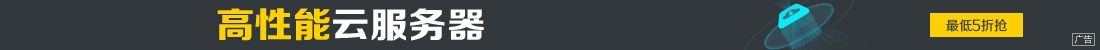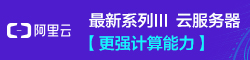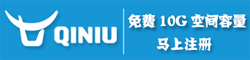# python机器学习之神经网络实现

••Python 的实现：

```model.add(Dense(1, input_dim=n_features, activation='linear', use_bias=True))

# Use mean squared error for the loss metric and use the ADAM backprop algorithm

# Train the network (learn the weights)
# We need to convert from DataFrame to NumpyArray
history = model.fit(X_train.values, y_train.values, epochs=100,
batch_size=1, verbose=2, validation_split=0)

```

```# create model
model = Sequential()
# Softmax output layer

model.fit(X_train.values, y_train.values, epochs=20, batch_size=16)

y_pred = model.predict(X_test.values)

y_te = np.argmax(y_test.values, axis = 1)
y_pr = np.argmax(y_pred, axis = 1)

print(np.unique(y_pr))

print(classification_report(y_te, y_pr))

print(confusion_matrix(y_te, y_pr))```

```from sklearn.model_selection import GridSearchCV

def create_model(optimizer='rmsprop'):
model = Sequential()
model.compile(loss='categorical_crossentropy', optimizer=optimizer, metrics=['accuracy'])

return model

model = KerasClassifier(build_fn=create_model, verbose=0)

optimizers = ['rmsprop']
epochs = [5, 10, 15]
batches = 

param_grid = dict(optimizer=optimizers, epochs=epochs, batch_size=batches, verbose=['2'])
grid = GridSearchCV(estimator=model, param_grid=param_grid)

grid.fit(X_train.values, y_train.values)
```